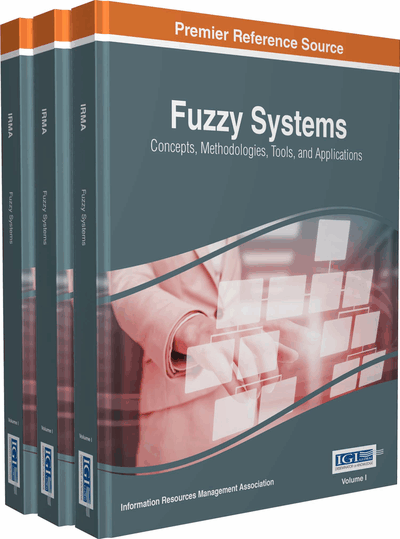# PSK Method for Solving Type-1 and Type-3 Fuzzy Transportation Problems

P. Senthil Kumar (Jamal Mohamed College (Autonomous), India)
DOI: 10.4018/978-1-5225-1908-9.ch017
Available
\$37.50
No Current Special Offers

## Abstract

In conventional transportation problem (TP), supplies, demands and costs are always certain. In this paper, the author tried to categories the TP under the mixture of certain and uncertain environment and formulates the problem and utilizes the crisp numbers, triangular fuzzy numbers (TFNs) and trapezoidal fuzzy numbers (TrFNs) to solve the TP. The existing ranking procedure of Liou and Wang is used to transform the type-1 and type-3 fuzzy transportation problem (FTP) into a crisp one so that the conventional method may be applied to solve the TP. The solution procedure differs from TP to type-1 and type-3 FTP in allocation step only. Therefore, the new method called PSK method and new multiplication operation on TrFN is proposed to find the mixed optimal solution in terms of crisp numbers, TFNs and TrFNs. The main advantage of this method is computationally very simple, easy to understand and also the optimum objective value obtained by our method is physically meaningful. The effectiveness of the proposed method is illustrated by means of a numerical example.
Chapter Preview
Top

## 1. Introduction

The transportation problem is a special class of linear programming problem which deals with the distribution of single homogeneous product from various origins(sources) to various destinations(sinks).The objective of the transportation problem is to determine the optimal amount of a commodity to be transported from various supply points to various demand points so that the total transportation cost is minimum for a minimization problem or total transportation profit is maximum for a maximization problem.

The unit costs, that is, the cost of transporting one unit from a particular supply point to a particular demand point, the amounts available at the supply points and the amounts required at the demand points are the parameters of the transportation problem. Efficient algorithms have been developed for solving transportation problems when the cost coefficients, the demand and supply quantities are known precisely.

In the history of mathematics, Hitchcock (1941) originally developed the basic transportation problem. Charnes and Cooper (1954) developed the stepping stone method which provides an alternative way of determining the simplex method information. Appa (1973) discussed several variations of the transportation problem. Arsham et al. (1989) proposed a simplex type algorithm for general transportation problems. An Introduction to Operations Research Taha (2008) deals the transportation problem.

In today’s real world problems such as in corporate or in industry many of the distribution problems are imprecise in nature due to variations in the parameters. To deal quantitatively with imprecise information in making decision, Zadeh (1965) introduced the fuzzy set theory and has applied it successfully in various fields. The use of fuzzy set theory becomes very rapid in the field of optimization after the pioneering work done by Bellman and Zadeh (1970). The fuzzy set deals with the degree of membership (belongingness) of an element in the set. In a fuzzy set the membership value (level of acceptance or level of satisfaction) lies between 0 and 1 where as in crisp set the element belongs to the set represent 1 and the element not belongs to the set represent 0.

Due to the applications of fuzzy set theory, several authors like Oheigeartaigh (1982) presented an algorithm for solving transportation problems where the availabilities and requirements are fuzzy sets with linear or triangular membership functions. Chanas et al. (1984) presented a fuzzy linear programming model for solving transportation problems with fuzzy supply, fuzzy demand and crisp costs. Chanas et al. (1993) formulated the fuzzy transportation problems in three different situations and proposed method for solving the formulated fuzzy transportation problems. Chanas and Kuchta (1996) proposed the concept of the optimal solution for the transportation problem with fuzzy coefficients expressed as fuzzy numbers, and developed an algorithm for obtaining the optimal solution.

Chanas and Kuchta (1998) developed a new method for solving fuzzy integer transportation problem by representing the supply and demand parameters as L-R type fuzzy numbers. Saad and Abbas (2003) proposed an algorithm for solving the transportation problems under fuzzy environment. Liu and Kao (2004) presented a method for solving fuzzy transportation problems based on extension principle. Chiang (2005) proposed a method to find the optimal solution of transportation problems with fuzzy requirements and fuzzy availabilities. Gani and Razak (2006) obtained a fuzzy solution for a two stage cost minimizing fuzzy transportation problem in which availabilities and requirements are trapezoidal fuzzy numbers using a parametric approach. Das and Baruah (2007) discussed Vogel’s approximation method to find the fuzzy initial basic feasible solution of fuzzy transportation problem in which all the parameters (supply, demand and cost) are represented by triangular fuzzy numbers. Li et al. (2008) proposed a new method based on goal programming approach for solving fuzzy transportation problems with fuzzy costs.

## Complete Chapter List

Search this Book:
Reset